# 3D Cartesian Coordinate Rotation (Euler) VI

## LabVIEW 2018 Help

Edition Date: March 2018
Part Number: 371361R-01
View Product InfoLabVIEW 2016 HelpLabVIEW 2017 HelpLabVIEW 2018 HelpLabVIEW 2019 HelpLabVIEW 2020 Help

Owning Palette: Geometry VIs

Requires: Full Development System

Rotates a three-dimensional Cartesian coordinate in the counterclockwise direction using the Euler angles method. Wire data to the X input to determine the polymorphic instance to use or manually select the instance.

Use the pull-down menu to select an instance of this VI.

 Select an instance 3D Cartesian Coordinate Rotation (Euler) (Array)3D Cartesian Coordinate Rotation (Euler) (Scalar)

## 3D Cartesian Coordinate Rotation (Euler) (Array)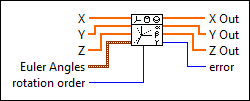X specifies the input x-coordinates.Y specifies the input y-coordinates.Z specifies the input z-coordinates.Euler Angles specifies the Euler angles in radians.phi specifies the first rotation angle about the first axis in radians.theta specifies the rotation angle about the second axis in radians.psi specifies the second rotation angle about the third axis in radians.rotation order specifies the order of the axes to rotate the coordinates around. For example, X-Y-Z specifies the first, second, and third rotations are about the x-, y-, and z-axes respectively. Z-X-Z is the default order.

 0 X-Y-Z 1 X-Z-Y 2 Y-X-Z 3 Y-Z-X 4 Z-X-Y 5 Z-Y-X 6 X-Y-X 7 X-Z-X 8 Y-X-Y 9 Y-Z-Y 10 Z-X-Z 11 Z-Y-Z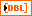X Out returns the rotated x-coordinates.Y Out returns the rotated y-coordinates.Z Out returns the rotated z-coordinates.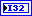error returns any error or warning from the VI. You can wire error to the Error Cluster From Error Code VI to convert the error code or warning into an error cluster.

## 3D Cartesian Coordinate Rotation (Euler) (Scalar)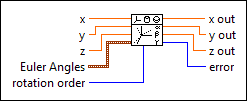x is the real input x-component for the two-element vector.y is the real input y-component for the two-element vector.z specifies the input z-coordinate.Euler Angles specifies the Euler angles in radians.phi specifies the first rotation angle about the first axis in radians.theta specifies the rotation angle about the second axis in radians.psi specifies the second rotation angle about the third axis in radians.rotation order specifies the order of the axes to rotate the coordinates around. For example, X-Y-Z specifies the first, second, and third rotations are about the x-, y-, and z-axes respectively. Z-X-Z is the default order.

 0 X-Y-Z 1 X-Z-Y 2 Y-X-Z 3 Y-Z-X 4 Z-X-Y 5 Z-Y-X 6 X-Y-X 7 X-Z-X 8 Y-X-Y 9 Y-Z-Y 10 Z-X-Z 11 Z-Y-Z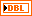x out returns the rotated x-coordinate.y out returns the rotated y-coordinate.z out returns the rotated z-coordinate.error returns any error or warning from the VI. You can wire error to the Error Cluster From Error Code VI to convert the error code or warning into an error cluster.

## 3D Cartesian Coordinate Rotation (Euler) Details

According to Euler's rotation theory, you can describe a coordinate rotation with the Euler angles,, and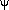as shown below (use the default rotation order Z-X-Z as an example):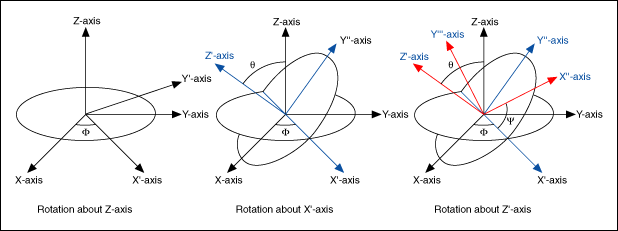The following three steps describe the rotation:

1. Rotate the X-, Y-, and Z-axes about the Z-axis by(–<), resulting in the X'-, Y'-, and Z-axes.
2. Rotate the X'-, Y'-, and Z-axes about the X'-axis by(0), resulting in the X'-, Y''-, and Z'-axes.
3. Rotate the X'-, Y''-, and Z'-axes about the Z'-axis by(–<), resulting in the X''-, Y'''-, and Z'-axes.

If you express the rotation of point (x, y, z) in terms of the following three matrices B, C, and D: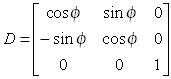;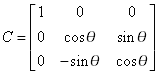;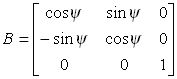,

the coordinates, (x', y', z'), of the point in the new coordinate frame are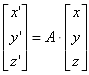where A = BCD.